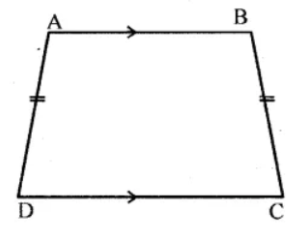QuestionAnswers

# If $ABCD$ is an isosceles trapezium, $\angle C$ is equal to ___________.A) $\angle B$B) $\angle A$C) $\angle D$D) $90^\circ$

Hint: We need to find the $\angle C$. So, we will check the properties of an isosceles trapezium. We will construct a figure of trapezium $ABCD$ and as in an isosceles trapezium, the base angles are always equal. So, we will construct the figure and name the angles accordingly and then check what is the other base angle with $\angle C$. Hence, it depends on how we name an isosceles trapezium. Thus, by this, we can find that $\angle C$ will be equal to.

Complete step by step solution: We will first construct an isosceles trapezium and name it as $ABCD$.As we know that an isosceles trapezium has a pair of opposite sides that are congruent.
Therefore, $AD$ is congruent to $BC$.
Since we need to find the value of $\angle C$, and as both pairs of opposite angles are supplementary that is they sum to $180^\circ$.
Thus, $\angle C + \angle D = 180$
Also, consecutive angles along both bases are congruent.
Hence, $\angle C$ and $\angle D$ are the base angles and base angles are always equal to each other.
Thus, we get that $\angle C = \angle D$.

Therefore, option C is correct.

Note: We need to remember the properties of an isosceles trapezium and construction of an isosceles trapezium is important. In an isosceles trapezium, consecutive angles along bases are congruent, diagonals are congruent hence, the base angles are always equal.